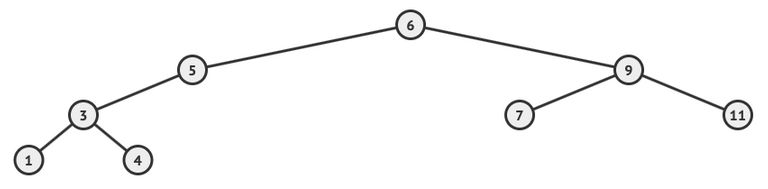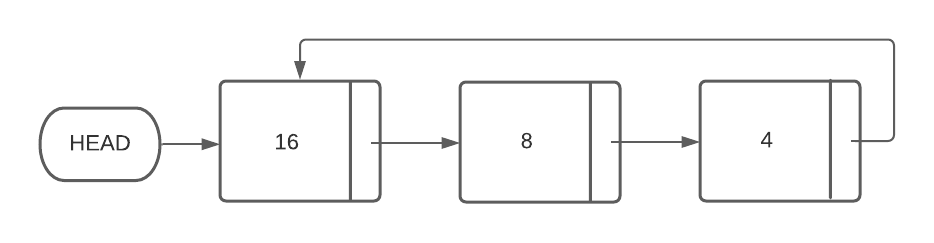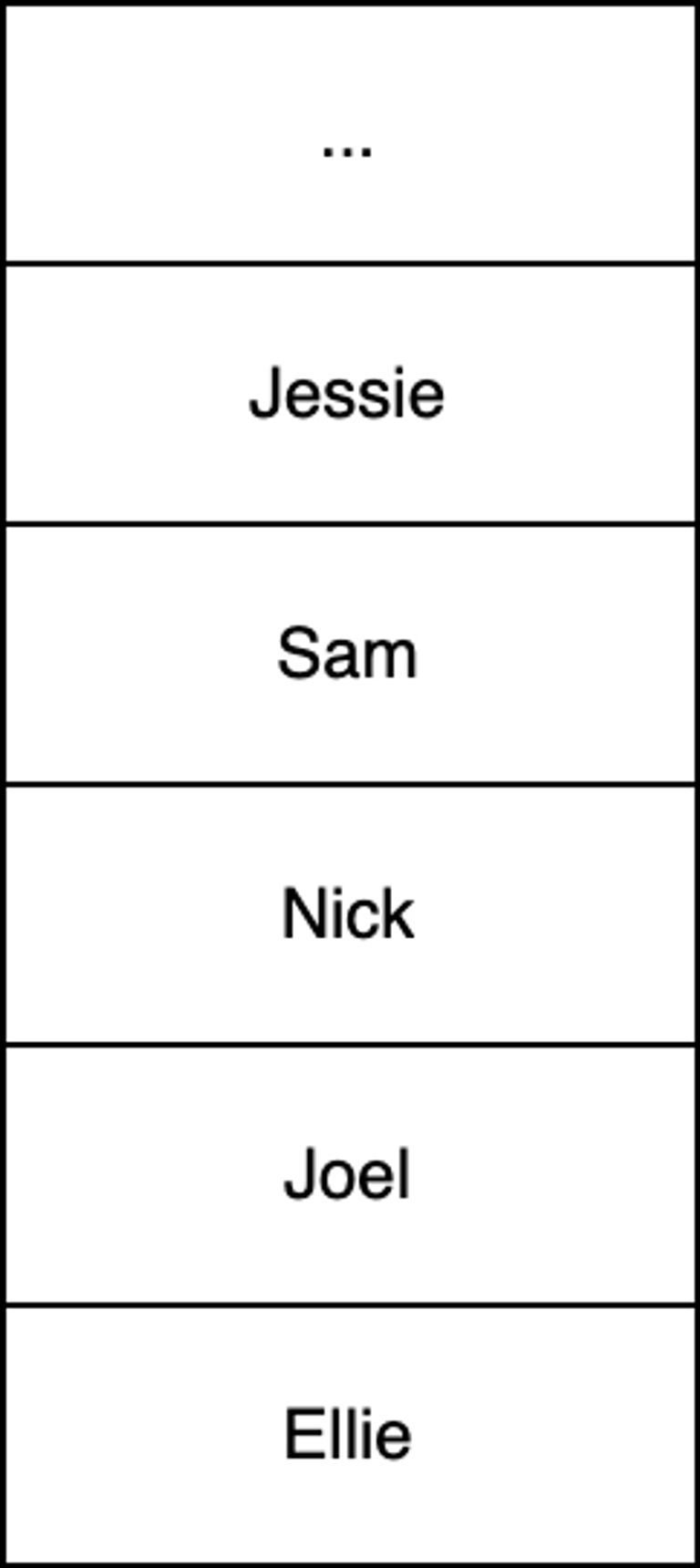Login or Create an Account to view question mark schemes, comment, and download a PDF of this test
Question 1[1 Mark]
What are the four basic functions of a computer
(a).
Process, input, output, store
(b).
Iterate, compare, output, store
(c).
Display, input, output, store
(d).
Input, output, process, search
Question 2[1 Mark]
Choose which of the following is not a form of system installation process.
(a).
Legacy changeover
(b).
Pilot running
(c).
Direct changeover
(d).
Parallel Running
Question 3[1 Mark]
Choose which one of the following testing sequences, in order from left to right, are typical of the software development cycle?
(a).
Unit Testing, Integration Testing, Alpha Testing, Beta Testing
(b).
Unit Testing, Alpha Testing, Beta Testing, Integration Testing
(c).
Alpha Testing, Unit Testing, Beta Testing, Integration Testing
(d).
Unit Testing, Alpha Testing, Integration Testing, Beta Testing
Question 4[1 Mark]
State the result of two Bubble Sort iterations where elements are arranged in ascending order (from lowest to highest running left to right) on the array [12, 32, 26, 37, 9]
(a).
[9, 12, 26, 37, 32]
(b).
[12, 9, 26, 32, 37]
(c).
[12, 26, 9, 32, 37]
(d).
[9, 12, 26, 32, 37]
Question 5[1 Mark]
Which of the following memory components is not typically found in the CPU?
(a).
(b).
Mode Register (MR)
(c).
Memory Data Register (MDR)
(d).
L2 cache
Question 6[1 Mark]
With respect to the concept of abstraction, which of the following statements is not true?
(a).
A city rail map is an abstraction of the city's rail system
(b).
A model airplane is an abstraction of the plane it represents
(c).
A city skyscraper is an abstraction of the building's floor plan
(d).
A mathematical function describing only the height of a bouncing ball is an abstraction of the ball's bouncing motion
Question 7[1 Mark]
If one ASCII character requires 8 bits of memory, how many characters can be stored in 4 bytes?
(a).
4 characters
(b).
8 characters
(c).
12 characters
(d).
2 characters
Question 8[1 Mark]
Choose the one option below which is not a resource that needs to be managed by the operating system.
(a).
Primary memory
(b).
Kernel
(c).
Screen resolution
(d).
Disk space
Question 9[1 Mark]
How many hexadecimal characters would be required to represent 2 bytes.
(a).
1
(b).
4
(c).
8
(d).
16
Question 10[1 Mark]
A circular queue implementation uses an array of length SIZE to hold queue items as well as two variables called REAR and FRONT to hold the current index positions of the rear (tail) and front (head) of the queue respectively. Enqueue and dequeue increment REAR and FRONT respectively. Select the conditional statement below which would, upon evaluating to True, identify the queue as full.
(a).
REAR = (FRONT + 1) % SIZE
(b).
FRONT = (REAR + 1) % SIZE
(c).
REAR + 1 = FRONT % SIZE
(d).
FRONT + 1 = REAR % SIZE
Question 11[2 Marks]
State two methods by which one can obtain system requirements from stakeholders
Question 12[5 Marks]
A clothes store currently uses the internal hard drive of a computer to store all their sales data.
(a).
Outline one example of how data loss issues may occur for the store

(b).
Describe two methods that the store could use to prevent data loss

Question 13[2 Marks]
Outline the primary function of the Memory Address Register (MAR) in the machine execution cycle.
Question 14[4 Marks]
Outline the steps of the Machine Instruction Cycle
Question 15[3 Marks]
Explain the characteristics of cache memory
Question 16[3 Marks]
Write a program that reads water temperature in degrees Celsius and outputs a message stating whether the water is frozen (less than zero degrees Celsius), boiling (greater than 100 degrees Celsius) or neither.
Question 17[11 Marks](a).
With respect to the binary search tree above, list all the nodes values according to the order in which they are visited by the following tree traversals.
(i).
In-order

(ii).
Pre-order

(iii).
Post-order

(b).
Describe how the post-order tree traversal algorithm works.

(c).
In contrast to arrays, outline two benefits of using binary search trees.

(d).
In contrast to a balanced binary search tree, identify one disadvantage of an unbalanced binary search tree;

Question 18[6 Marks]
(a).
State what is meant by the term ‘dynamic data structure’

Consider the following circularly linked list(b).
Given a new node containing the number 7 (called NEW_NODE) and a pointer (called PREV_NODE) which points to the node containing the number 8, describe how the new node should be added to the linked list in such a way that the circularly linked list remains both properly formed and sorted.

(c).
In contrast to the process described in part b), outline why it would be less efficient to add the value 32 to a static array containing [16, 8, 4] in such as way as to preserve both existing values and ascending order.

Question 19[12 Marks]
At the moment marathon runners cross the finishing line of a race, their names are added to a stack called RUNNERS.
For example, a diagram of RUNNERS below indicates “Ellie” has finished first and “Jessie” last. “Jessie” is at the top of the RUNNERS stack.(a).
Using pseudocode, construct an efficient procedure that will search the stack RUNNERS for a name given via standard input. Once the given name is found, output the finishing place of the runner specified as a number. If a name is not found then the procedure should output the message "Runner not found". Assume all names are unique, the number of elements in RUNNERS is unknown and RUNNERS may be emptied during the search process.

For example, given an input string of "Joel" the procedure will output the number 2 to indicate Joel finished in 2nd place.
(b).
Outline why the use of a queue would make this algorithm simpler and more efficient than using a stack.

In the same way as RUNNERS saves each runner's name, another stack called TIMES saves each runner's finishing time as they cross the finish line.
(c).
The results of a particular competition where 101 runners successfully finish a race are held in RUNNERS and TIMES. Given an empty 101x2 (row x column) 2D array called RESULTS, write pseudocode such that the first column contains the names of all those runners who finished the race and the second column contains their respective times in ascending rank order.

For example, RESULTS should contain the time of the runner who finished in 94th place, that is, having only ran faster than seven people (eighth last).
(d).
Using RESULTS, state the single line of pseudocode that represents the name of the runner with the median finishing time.


HomeThe Right Test - Fast